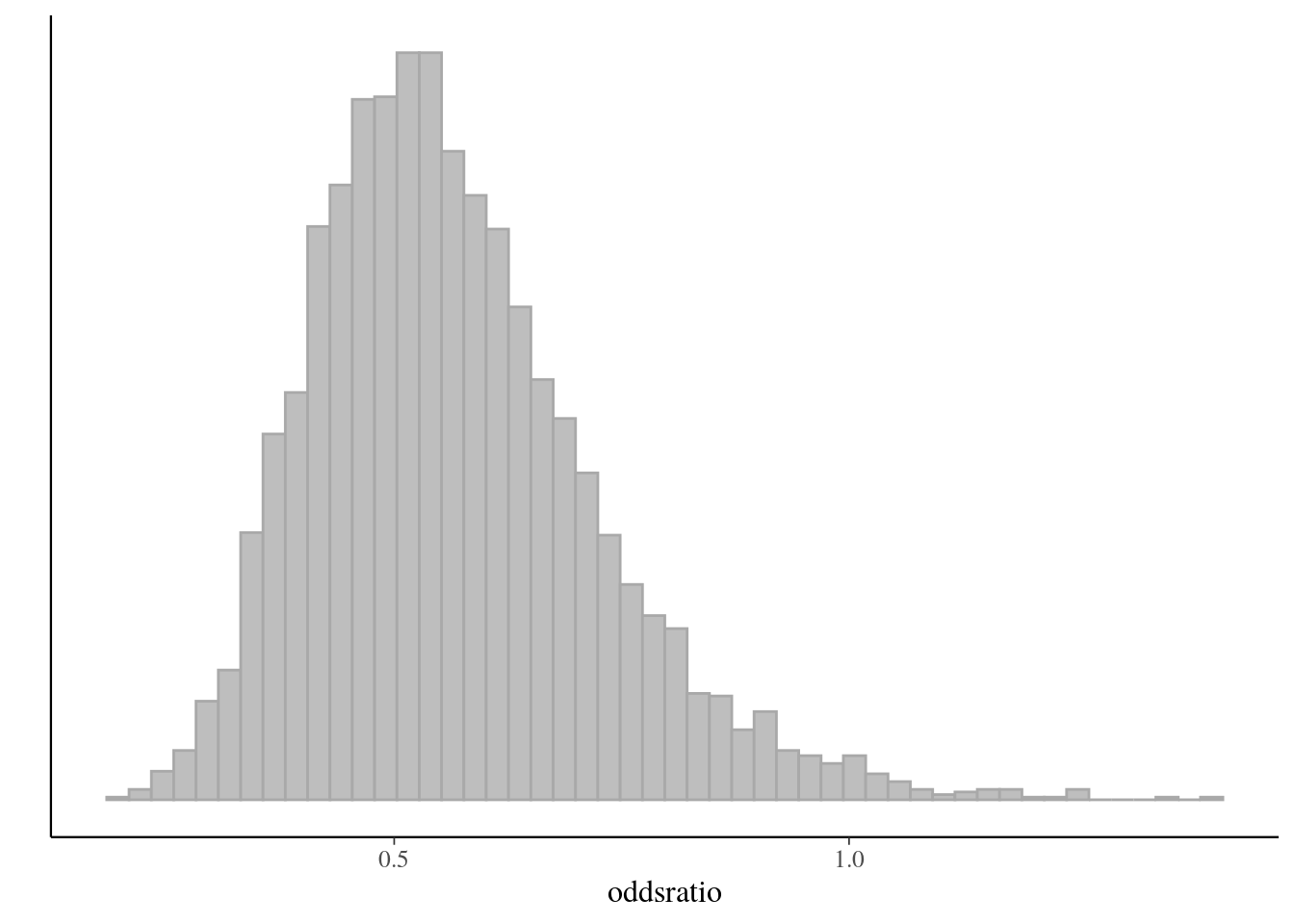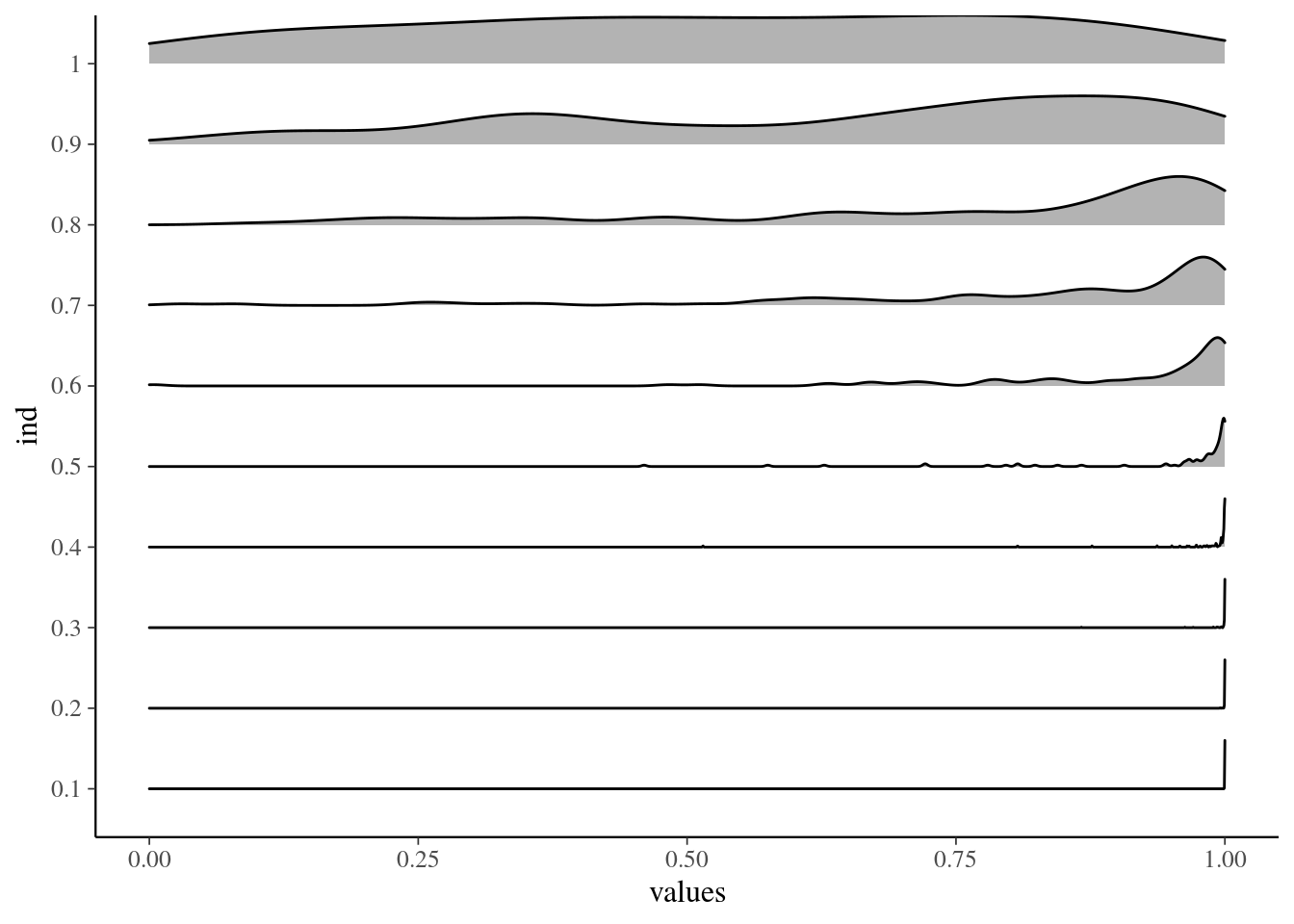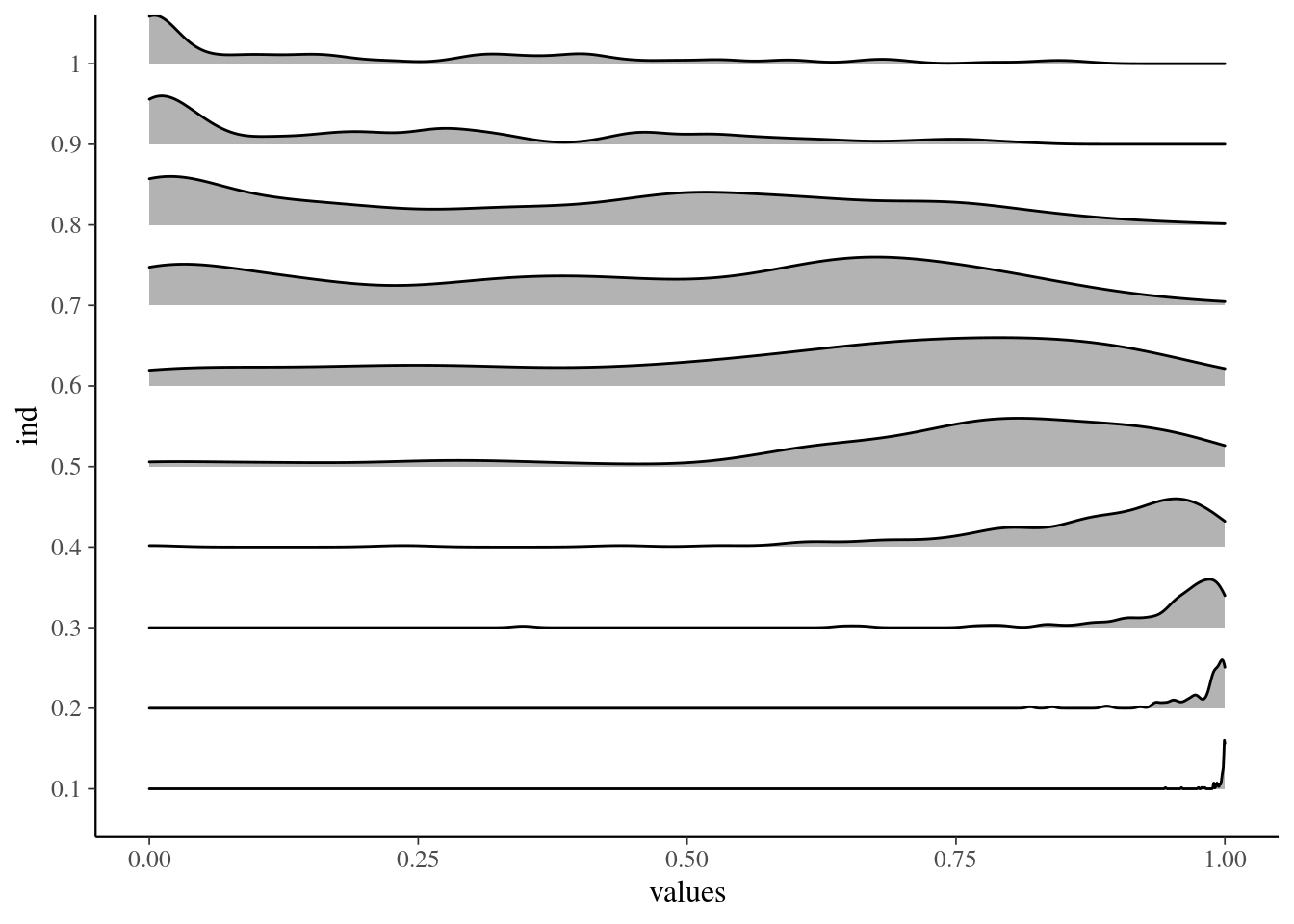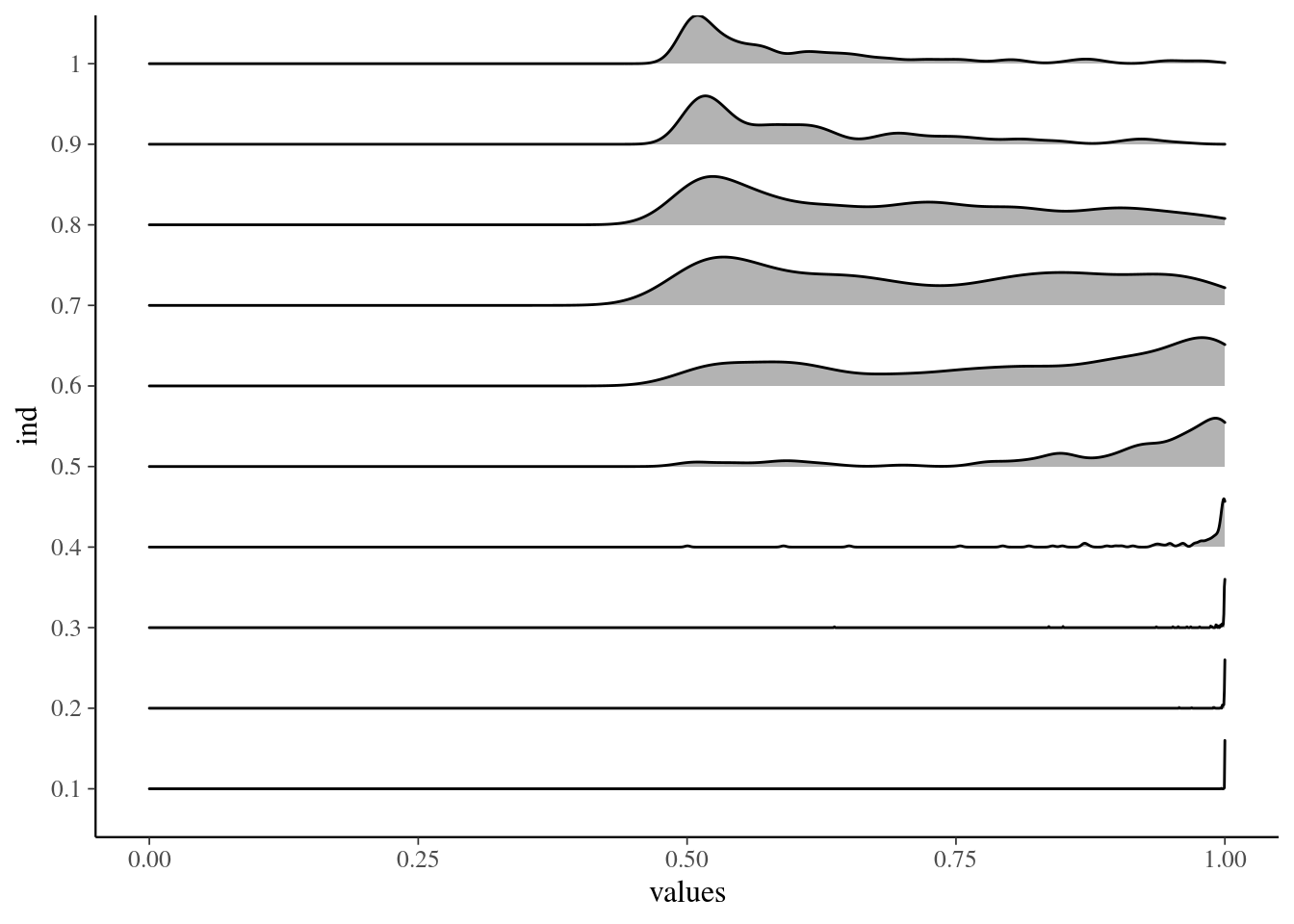# 1 Setup

library(tidyr)
library(rstanarm)
library(loo)
library(ggplot2)
theme_set(bayesplot::theme_default())
library(ggridges)
library(bridgesampling)

# 2 Introduction

This notebook demonstrates a simple model we trust, that is, we assume $$M$$-closed case (Vehtari and Ojanen, 2012). In this case, cross-validation is not needed and we we can get better accuracy using the explicit model.

# 3 Comparison of two groups with Binomial

An experiment was performed to estimate the effect of beta-blockers on mortality of cardiac patients (the example is from Gelman et al., 2013, Ch 3). A group of patients were randomly assigned to treatment and control groups:

• out of 674 patients receiving the control, 39 died
• out of 680 receiving the treatment, 22 died

Data, where grp2 is a dummy variable that captures the difference of the intercepts in the first and the second group.

d_bin2 <- data.frame(N = c(674, 680), y = c(39,22), grp2 = c(0,1))

## 3.1 Analysis of the observed data

To analyse whether the treatment is useful, we can use Binomial model for both groups and compute odds-ratio.

fit_bin2 <- stan_glm(y/N ~ grp2, family = binomial(), data = d_bin2,
weights = N, refresh=0)

In general we recommend showing the full posterior of the quantity of interest, which in this case is the odds ratio.

samples_bin2 <- rstan::extract(fit_bin2$stanfit) theta1 <- plogis(samples_bin2$alpha)
theta2 <- plogis(samples_bin2$alpha + samples_bin2$beta)
oddsratio <- (theta2/(1-theta2))/(theta1/(1-theta1))
ggplot() + geom_histogram(aes(oddsratio), bins = 50, fill = 'grey', color = 'darkgrey') +
labs(y = '') + scale_y_continuous(breaks = NULL)The probability that odds-ratio is less than 1:

print(mean(oddsratio<1),2)
 0.99

This posterior distribution of the odds-ratio (or some transformation of it) is the simplest and the most accurate way to analyse the effectiveness of the treatment. In this case, there is high probability that the treatment is effective and relatively big.

## 3.2 Simulation experiment

Although we recommend showing the full posterior, the probability that oddsratio < 1 can be a useful summary. Simulation experiment binom_odds_comparison.R runs 100 simulations with simulated data with varying oddsratio (0.1,…,1.0) and computes for each run the probability that oddsratio<1. The following figures show the variation in the results.

Variation in probability that oddsratio<1 when true oddsratio is varied.

load(file="binom_test_densities.RData")
ggplot(betaprobs_densities, aes(x = values, y = ind, height = scaled)) +
geom_density_ridges(stat = "identity", scale=0.6)# 4 Cross-validation

Sometimes it is better to focus on observable space (we can’t observe $$\theta$$ or odds-ratio directly, but we can observe $$y$$). In leave-one-out cross-validation, model is fitted $$n$$ times with each observation left out at time in fitting and used to evaluate the predictive performance. This corresponds to using the already seen observations as pseudo Monte Carlo samples from the future data distribution, with the leave-trick used to avoid double use of data. With the often used log-score we get $\mathrm{LOO} = \frac{1}{n} \sum_{i=1}^n \log {p(y_i|x_i,D_{-i},M_k)}$. Cross-validation is useful in $$M$$-open case (Vehtari and Ojanen, 2012), when we don’t trust any model to present the future data well (they might, but we just don’t have enough confidence to trust them to do so).

Next we demonstrate one of the weaknesses of cross-validation (same holds for WAIC etc.).

## 4.1 Analysis of the observed data

To use leave-one-out where “one” refers to an individual patient, we need to change the model formulation a bit. In the above model formulation, the individual observations have been aggregated to group observations and running loo(fit_bin2) would try to leave one group completely. In case of having more groups, this could be what we want, but in case of just two groups it is unlikely. Thus, in the following we switch a Bernoulli model with each individual as it’s own observation.

d_bin2b <- data.frame(y = c(rep(1,39), rep(0,674-39), rep(1,22), rep(0,680-22)), grp2 = c(rep(0, 674), rep(1, 680)))
fit_bin2b <- stan_glm(y ~ grp2, family = binomial(), data = d_bin2b, seed=180202538, refresh=0)

We fit also a “null” model which doesn’t use the group variable and thus has common parameter for both groups.’

fit_bin2bnull <- stan_glm(y ~ 1, family = binomial(), data = d_bin2b, seed=180202538, refresh=0)

We can then use cross-validation to compare whether adding the treatment variable improves predictive performance. We use fast Pareto smoothed importance sampling leave-one-out cross-validation (PSIS-LOO; Vehtari, Gelman and Gabry, 2017b).

(loo_bin2 <- loo(fit_bin2b))

Computed from 4000 by 1354 log-likelihood matrix

Estimate   SE
elpd_loo   -248.1 23.4
p_loo         2.0  0.3
looic       496.3 46.7
------
Monte Carlo SE of elpd_loo is 0.0.

All Pareto k estimates are good (k < 0.5).
See help('pareto-k-diagnostic') for details.
(loo_bin2null <- loo(fit_bin2bnull))

Computed from 4000 by 1354 log-likelihood matrix

Estimate   SE
elpd_loo   -249.7 23.4
p_loo         1.0  0.1
looic       499.4 46.7
------
Monte Carlo SE of elpd_loo is 0.0.

All Pareto k estimates are good (k < 0.5).
See help('pareto-k-diagnostic') for details.

All Pareto $$k<0.5$$ and we can trust PSIS-LOO result (Vehtari, Gelman and Gabry, 2017b, 2017a).

Let’s make pairwise comparison.

loo_compare(loo_bin2null, loo_bin2)
              elpd_diff se_diff
fit_bin2b      0.0       0.0
fit_bin2bnull -1.5       2.3   

elpd_diff is small compared to se, and thus cross-validation is uncertain whether estimating the treatment effect improves the predictive performance. To put this in perspective, we have $$N_1=674$$ and $$N_2=680$$, and 5.8% and 3.2% deaths, and this is now too weak information for cross-validation.

## 4.2 Simulation experiment

Simulation experiment binom_odds_comparison.R runs 100 simulations with simulated data with varying oddsratio (0.1,…,1.0) and computes LOO comparison for each run.

Variation in LOO comparison when true oddsratio is varied.

ggplot(looprobs_densities, aes(x = values, y = ind, height = scaled)) +
geom_density_ridges(stat = "identity", scale=0.6)We see that using the posterior distribution from the model is more efficient to detect the effect, but cross-validation will detect it eventually too. The difference here comes that cross-validation doesn’t trust the model, compares the model predictions to the “future data” using very weak assumption about the future. The weak assumption about the future is also the cross-validation strength as we we’ll see in another notebook.

# 5 Reference predictive approach

We can also do predictive performance estimates using stronger assumption about the future. A reference predictive estimate with log-score can be computed as $\mathrm{elpd}_{\mathrm{ref}} = \int p(\tilde{y}|D,M_*) \log p(\tilde{y}|D,M_k) d\tilde{y},$ where $$M_*$$ is a reference model we trust. Using a reference model to assess the other models corresponds to $$M$$-completed case (Vehtari and Ojanen, 2012), where the true model is replaced with a model we trust to be close enough to the true model.

## 5.1 Simulation experiment

The next figure shows the results from the same simulation study using a reference predictive approach with the fit_bin2 model used as the reference.

ggplot(refprobs_densities, aes(x = values, y = ind, height = scaled)) +
geom_density_ridges(stat = "identity", scale=0.6)We can see better accuracy than for cross-validation. The similar improvement in the model selection performance is observed in projection predictive variable selection (Piironen and Vehtari, 2017; Piironen, Paasiniemi and Vehtari, 2018) implemented in projpred package.

# 6 Marginal likelihood

As comparison we include marginal likelihood based approach to compute the posterior probabilities for the null model (treatment effect is zero) and the model with unknown treatment effect. As the data and models are very simple, we may assume $$M$$-closed case. Marginal likelihoods and relative posterior probabilities can be sensitive to selected prior on the more complex model. Here we simply use the same prior as for the above examples. Marginal likelihoods are computed using the default bridge sampling approach implemented in bridge_sampling package.

## 6.1 Analysis of the observed data

# rerun models with diagnostic file required by bridge_sampler
fit_bin2 <- stan_glm(y/N ~ grp2, family = binomial(), data = d_bin2,
weights = N, refresh=0,
diagnostic_file = file.path(tempdir(), "df.csv"))
(ml_bin2 <- bridge_sampler(fit_bin2, silent=TRUE))
Bridge sampling estimate of the log marginal likelihood: -11.80549
Estimate obtained in 4 iteration(s) via method "normal".
fit_bin2null <- stan_glm(y/N ~ 1, family = binomial(), data = d_bin2,
weights = N, refresh=0,
diagnostic_file = file.path(tempdir(), "df.csv"))
(ml_bin2null <- bridge_sampler(fit_bin2null, silent=TRUE))
Bridge sampling estimate of the log marginal likelihood: -12.14588
Estimate obtained in 5 iteration(s) via method "normal".
print(post_prob(ml_bin2, ml_bin2null), digits=2)
    ml_bin2 ml_bin2null
0.58        0.42 

Posterior probability computed from the marginal likelihoods is indecisive.

## 6.2 Simulation experiment

We repeat the simulation with marginal likelihood approach.

ggplot(bfprobs_densities, aes(x = values, y = ind, height = scaled)) +
geom_density_ridges(stat = "identity", scale=0.6)